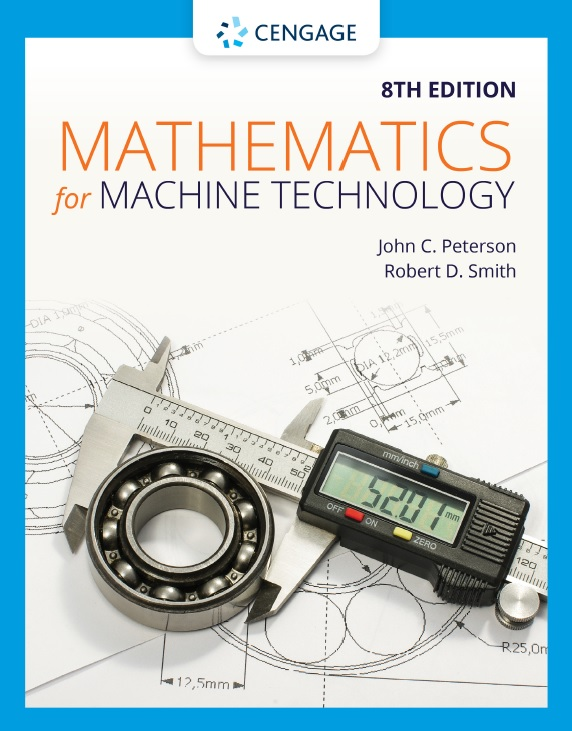# Mathematics for Machine Technology, 8th Edition PDF by John C Peterson and Robert D Smith

## Mathematics for Machine Technology, Eighth Edition

By John C. Peterson and Robert D. SmithContents

vi Preface

1 section 1 Common Fractions and Decimal Fractions

UNIT 1 Introduction to Common Fractions and Mixed Numbers / 1

UNIT 2 Addition of Common Fractions and Mixed Numbers / 8

UNIT 3 Subtraction of Common Fractions and Mixed Numbers / 14

UNIT 4 Multiplication of Common Fractions and Mixed Numbers / 20

UNIT 5 Division of Common Fractions and Mixed Numbers / 27

UNIT 6 Combined Operations of Common Fractions and Mixed Numbers / 33

UNIT 7 Computing with a Calculator: Fractions and Mixed Numbers / 40

UNIT 8 Computing with a Spreadsheet: Fractions and Mixed Numbers / 49

UNIT 9 Introduction to Decimal Fractions / 57

UNIT 10 Rounding Decimal Fractions and Equivalent Decimal and Common Fractions / 63

UNIT 11 Addition and Subtraction of Decimal Fractions / 69

UNIT 12 Multiplication of Decimal Fractions / 74

UNIT 13 Division of Decimal Fractions / 78

UNIT 14 Powers / 84

UNIT 15 Roots / 91

UNIT 16 Table of Decimal Equivalents and Combined Operations of Decimal Fractions / 97

UNIT 17 Computing with a Calculator: Decimals / 103

UNIT 18 Computing with a Spreadsheet: Decimals / 112

UNIT 19 Achievement Review—Section One / 121

126 Section 2 Ratio, Proportion, and Percentage

UNIT 20 Ratio and Proportion / 126

UNIT 21 Direct and Inverse Proportions / 135

UNIT 22 Introduction to Percents / 141

UNIT 23 Basic Calculations of Percentages, Percents, and Rates / 145

UNIT 24 Percent Practical Applications / 150

UNIT 25 Achievement Review—Section Two / 157

160 Section 3 Linear Measurement: Customary (English) and Metric

UNIT 26 Customary (English) Units of Measure / 160

UNIT 27 Metric Units of Linear Measure / 169

UNIT 28 Degree of Precision, Greatest Possible Error, Absolute Error, and Relative Error / 178

UNIT 29 Tolerance, Clearance, and Interference / 183

UNIT 30 Customary and Metric Steel Rules / 192

UNIT 31 Customary Vernier Calipers and Height Gages / 203

UNIT 32 Metric Vernier Calipers and Height Gages / 212

UNIT 33 Digital Calipers and Height Gages / 215

UNIT 34 Customary Micrometers / 218

UNIT 35 Metric Vernier Micrometers / 226

UNIT 36 Digital Micrometers / 231

UNIT 37 Customary and Metric Gage Blocks / 234

UNIT 38 Achievement Review—Section Three / 238

244 Section 4 Fundamentals of Algebra

UNIT 39 Symbolism and Algebraic Expressions / 244

UNIT 40 Signed Numbers / 253

UNIT 41 Algebraic Operations of Addition, Subtraction, and Multiplication / 267

UNIT 42 Algebraic Operations of Division, Powers, and Roots / 274

UNIT 43 Introduction to Equations / 287

UNIT 44 Solution of Equations by the Subtraction, Addition, and Division Principles of Equality / 295

UNIT 45 Solution of Equations by the Multiplication, Root, and Power Principles of Equality / 306

UNIT 46 Solution of Equations Consisting of Combined Operations and Rearrangement of Formulas / 313

UNIT 47 Applications of Formulas to Cutting Speed, Revolutions per Minute, and Cutting Time / 324

UNIT 48 Applications of Formulas to Spur Gears / 334

UNIT 49 Achievement Review—Section Four / 343

347 Section 5 Fundamentals of Plane Geometry

UNIT 50 Lines and Angular Measure / 347

UNIT 51 Protractors—Simple Semicircular and Vernier / 363

UNIT 52 Types of Angles and Angular Geometric Principles / 370

UNIT 53 Introduction to Triangles / 380

UNIT 54 Geometric Principles for Triangles and Other Common Polygons / 387

UNIT 55 Introduction to Circles / 399

UNIT 56 Arcs and Angles of Circles, Tangent Circles / 409

UNIT 57 Fundamental Geometric Constructions / 420

UNIT 58 Achievement Review—Section Five / 430

437 Section 6 Geometric Figures: Areas and Volumes

UNIT 59 Areas of Rectangles, Parallelograms, and Trapezoids / 437

UNIT 60 Areas of Triangles / 449

UNIT 61 Areas of Circles, Sectors, and Segments / 454

UNIT 62 Volumes of Prisms and Cylinders / 462

UNIT 63 Volumes of Pyramids and Cones / 473

UNIT 64 Volumes of Spheres and Composite Solid Figures / 481

UNIT 65 Achievement Review—Section Six / 488

494 Section 7 Trigonometry

UNIT 66 Introduction to Trigonometric Functions / 494

UNIT 67 Analysis of Trigonometric Functions / 506

UNIT 68 Basic Calculations of Angles and Sides of Right Triangles / 511

UNIT 69 Simple Practical Machine Applications / 519

UNIT 70 Complex Practical Machine Applications / 529

UNIT 71 The Cartesian Coordinate System / 542

UNIT 72 Oblique Triangles: Law of Sines and Law of Cosines / 546

UNIT 73 Achievement Review—Section Seven / 558

563 Section 8 Compound Angles

UNIT 74 Introduction to Compound Angles / 563

UNIT 75 Drilling and Boring Compound-Angular Holes: Computing Angles of Rotation and Tilt Using Given Lengths / 567

UNIT 76 Drilling and Boring Compound-Angular Holes: Computing Angles of Rotation and Tilt Using Given Angles / 573

UNIT 77 Machining Compound-Angular Surfaces: Computing Angles of Rotation and Tilt / 581

UNIT 78 Computing Angles Made by the Intersection of Two Angular Surfaces / 590

UNIT 79 Computing Compound Angles on Cutting and Forming Tools / 598

UNIT 80 Achievement Review—Section Eight / 608

610 section 9 Computer Numerical Control (CNC)

UNIT 81 Introduction to Computer Numerical Control (CNC) / 610

UNIT 82 Control Systems, Absolute Positioning, Incremental Positioning / 621

UNIT 83 Location of Points: Polar Coordinate System / 631

UNIT 84 Binary Numeration System / 638

UNIT 85 Hexadecimal Numeration System / 645

UNIT 86 BCD (Binary Coded Decimal) Numeration Systems / 650

UNIT 87 An Introduction to G- and M-Codes for CNC Programming / 655

UNIT 88 Achievement Review—Section Nine / 669

674 Appendixes

A United States Customary and Metric Units of Measure / 674

B Principles of Plane Geometry / 676

C Formulas for Areas (A ) of Plane Figures / 678

D Formulas for Volumes (V ) of Solid Figures / 679

E Trigonometry / 680

F Common G- and M-Codes / 682